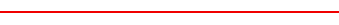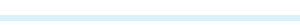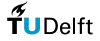A causal model for qualitative reasoningtitle: A causal model for qualitative reasoning author: Paul Maaskant published in: July 2006 appeared as: Master of Science thesis Man-machine interaction group Delft University of Technology PDF (2859 KB)### Abstract

Many problems can be solved by means of qualitative reasoning. When the complexity of a qualitative problem in an uncertain domain becomes nontrivial, the merit of a reasoning framework becomes apparent. Bayesian networks (BNs) provide such a network. BNs provide a concise way to represent a fully joint probability distribution, by exploiting independencies among variables. Each variable is specified by a Conditional Probability Table (CPT). A CPT captures the interaction between a child variable and its immediate predecessors (parents) variables. However, BNs are more quantitative in nature than qualitative, and thus a translation is required. Furthermore, a fundamental problem of BNs is the exponential growth of a CPT by the number of parent variables for a local probability distribution. A Causal interaction model, such as the Noisy-OR model, can provide a solution for this type of problem. The contribution of this thesis is the proposal of a causal model, baptized the Probabilistic Qualitative (PQ) model, that provides a reasoning framework for qualitative problems, in an uncertain domain. In this thesis, I review theory related to the PQ-model. I discuss the most prominent existing solution to our research problem, i.e., CAusal STrenght (CAST) logic. I give a detailed account of the theoretic PQ-model. I describe an experiment, conducted at the University of Pittsburgh, that proves the merits of the PQ-model, and I given an account of the software engineering project that led to the software application that captures the functionality of the PQ-model.This page was updated on July 05, 2006by webmaster@kbs